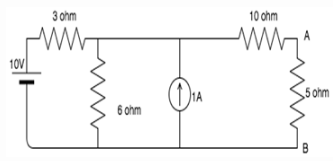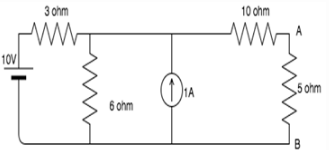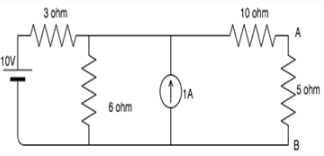Courses

# Test: Norton’s Theorem

## 10 Questions MCQ Test Networking Theory | Test: Norton’s Theorem

Description
This mock test of Test: Norton’s Theorem for Electrical Engineering (EE) helps you for every Electrical Engineering (EE) entrance exam. This contains 10 Multiple Choice Questions for Electrical Engineering (EE) Test: Norton’s Theorem (mcq) to study with solutions a complete question bank. The solved questions answers in this Test: Norton’s Theorem quiz give you a good mix of easy questions and tough questions. Electrical Engineering (EE) students definitely take this Test: Norton’s Theorem exercise for a better result in the exam. You can find other Test: Norton’s Theorem extra questions, long questions & short questions for Electrical Engineering (EE) on EduRev as well by searching above.
QUESTION: 1

### The Norton current is the_______

Solution:

Norton current is the short circuit current. It is the current through the specified load resistance. It is not the open circuit current because open circuit current is equal to zero.

QUESTION: 2

### Norton resistance is found by?

Solution:

Current sources have infinite internal resistance hence behave like an open circuit whereas ideal voltage sources have 0 internal resistances hence behave as a short circuit.

QUESTION: 3

### Norton’s theorem is true for __________

Solution:

Norton’s theorem works for only linear circuit elements and not non-linear ones such as BJT, semiconductors etc.

QUESTION: 4

In Norton’s theorem Isc is__________

Solution:

Norton’s theorem states that a combination of voltage sources, current sources and resistors is equivalent to a single current source Ith and a single parallel resistor R.

QUESTION: 5

Isc is found across the ____________ terminals of the network.

Solution:

According to Norton’s theorem, Isc is found through the output terminals of a network and not the input terminals.

QUESTION: 6

Can we use Norton’s theorem on a circuit containing a BJT?

Solution:

We can use Norton’s theorem only for linear networks. BJT is a non-linear network hence we cannot apply Norton’s theorem for it.

QUESTION: 7

Calculate the Norton’s equivalent voltage for the following circuit if 5 ohm is the load resistance.​Solution:

Shorting all voltage sources and opening all current sources we have:
RN=(3||6)+10= 12 ohm.

QUESTION: 8

Calculate the short circuit current is the 5 ohm resistor is the load resistance.​Solution:

Since the 5 ohm is the load resistance, we short it and find the resistance through the short.
If we apply source transformation between the 6 ohm resistor and the 1A source, we get a 6V source in series with a 6 ohm resistor. Now we have two meshes. Let us consider I1 flowing in the first mesh and I2 flowing in the second mesh.
The mesh equations are:
9I1-6I2=4
-6I1+16I2=6
On solving these equations simultaneously, we get I2=0.72A, which is the short circuit current.

QUESTION: 9

Find the current in the 5 ohm resistance using Norton’s theorem.​Solution:

From Q8 and Q7 we have found the values of the Isc and RN respectively.
Connecting the current source in parallel to RN which is in turn connected in parallel to the load resistance=5ohm, we get the Norton’s equivalent circuit.
Using current divider: I=0.72*12/(12+5)= 0.5 A.

QUESTION: 10

Which of the following is also known as the dual of Norton’s theorem?

Solution:

Thevenin’s theorem is also known as the dual of Norton’s theorem because in Norton’s theorem we find short circuit current which is the dual of open circuit voltage-what we find in Thevenin’s theorem.